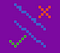# Better Line Tool for Pixel Art

Last week I went over how to implement flood fill and line tool in HTML Canvas with undo/redo functionality. However, I wasn’t happy with the line tool code because the lines had extra squares that I didn’t want to draw. The code I was using was originally for non-pixel art, so this time I reworked the code to make pixel-perfect lines.Top line is before, bottom line is after

First, let’s look at the old code:

`function actionLine(sx, sy, tx, ty, currentColor, ctx, scale = 1) {  ctx.fillStyle = currentColor.color;  // finds the distance between points  function lineLength(x1, y1, x2, y2) {    return Math.sqrt((x2 - x1) * (x2 - x1) + (y2 - y1) * (y2 - y1));  }  // finds the angle of (x,y) on a plane from the origin  function getAngle(x, y) {    return Math.atan(y / (x == 0 ? 0.01 : x)) + (x < 0 ? Math.PI : 0);  }  let dist = lineLength(sx, sy, tx, ty); // length of line  let ang = getAngle(tx - sx, ty - sy); // angle of line  for (let i = 0; i < dist; i++) {    // for each point along the line    ctx.fillRect(      Math.round(sx + Math.cos(ang) * i) * scale, // round for perfect pixels      Math.round(sy + Math.sin(ang) * i) * scale, // thus no aliasing      scale,      scale    ); // fill in one pixel, 1x1  }  //fill endpoint  ctx.fillRect(    Math.round(tx) * scale, // round for perfect pixels    Math.round(ty) * scale, // thus no aliasing    scale,    scale  ); // fill in one pixel, 1x1}`

One major problem with this code is that we’re iterating through points along the line (on the diagonal), however for pixel art there’s only as many pixels as either the vertical or horizontal component, depending on which one is longer. Also, instead of just taking the cosine for the x component, and sine for the y component, we’ll want just the length for the longer component. For the shorter component, we need to do a little bit more trigonometry.

`xComponent = sx + Math.sign(Math.cos(ang))*i;yComponent = sy + Math.tan(ang)*Math.sign(Math.cos(ang))*i;`

So for the longer component, we just need the sign of the cosine to draw in the correct direction. For the shorter component, we multiply by the tangent as well. However, this won’t work for angles that have a longer y component. This is because the side we’re calculating for is now adjacent, not opposite. To fix this, I chose to change which angle we’re using to calculate instead of using different trig functions.

`xComponent = Math.tan((Math.PI/2)-ang)*Math.sign(Math.cos((Math.PI/2)-ang));yComponent = Math.sign(Math.cos((Math.PI/2)-ang));`

Here’s the new code, which switches the calculation based on which component is longer:

`function actionLine(sx, sy, tx, ty, currentColor, ctx, scale = 1) {    ctx.fillStyle = currentColor.color;    //create triangle object    let tri = {}    function getTriangle(x1,y1,x2,y2,ang) {        if(Math.abs(x1-x2) > Math.abs(y1-y2)) {            tri.x = Math.sign(Math.cos(ang));            tri.y = Math.tan(ang)*Math.sign(Math.cos(ang));            tri.long = Math.abs(x1-x2);        } else {             tri.x = Math.tan((Math.PI/2)-ang)*Math.sign(Math.cos((Math.PI/2)-ang));            tri.y = Math.sign(Math.cos((Math.PI/2)-ang));            tri.long = Math.abs(y1-y2);        }    }    // finds the angle of (x,y) on a plane from the origin    function getAngle(x,y) { return Math.atan(y/(x==0?0.01:x))+(x<0?Math.PI:0); }let angle = getAngle(tx-sx,ty-sy); // angle of line    getTriangle(sx,sy,tx,ty, angle);    for(let i=0;i<tri.long;i++) {        let thispoint = {x: Math.round(sx + tri.x*i), y: Math.round(sy + tri.y*i)};        // for each point along the line        ctx.fillRect(thispoint.x*scale, // round for perfect pixels                    thispoint.y*scale, // thus no aliasing                    scale,scale); // fill in one pixel, 1x1    }    //fill endpoint    ctx.fillRect(Math.round(tx)*scale, // round for perfect pixels                    Math.round(ty)*scale, // thus no aliasing                    scale,scale); // fill in one pixel, 1x1}`